Test: Source Transformations

# Test: Source Transformations

Test Description

## 10 Questions MCQ Test Basic Electrical Technology | Test: Source Transformations

Test: Source Transformations for Electrical Engineering (EE) 2023 is part of Basic Electrical Technology preparation. The Test: Source Transformations questions and answers have been prepared according to the Electrical Engineering (EE) exam syllabus.The Test: Source Transformations MCQs are made for Electrical Engineering (EE) 2023 Exam. Find important definitions, questions, notes, meanings, examples, exercises, MCQs and online tests for Test: Source Transformations below.
Solutions of Test: Source Transformations questions in English are available as part of our Basic Electrical Technology for Electrical Engineering (EE) & Test: Source Transformations solutions in Hindi for Basic Electrical Technology course. Download more important topics, notes, lectures and mock test series for Electrical Engineering (EE) Exam by signing up for free. Attempt Test: Source Transformations | 10 questions in 10 minutes | Mock test for Electrical Engineering (EE) preparation | Free important questions MCQ to study Basic Electrical Technology for Electrical Engineering (EE) Exam | Download free PDF with solutions
 1 Crore+ students have signed up on EduRev. Have you?
Test: Source Transformations - Question 1

### A voltage source connected in series with a resistor can be converted to a?

Detailed Solution for Test: Source Transformations - Question 1

A voltage source connected in series can be converted to a current source connected in parallel using the relation obtained from ohm’s law, that is V=IR. This equation shows that a voltage source connected in series has the same impact as a current source connected in parallel.

Test: Source Transformations - Question 2

### Calculate the total current in the circuit.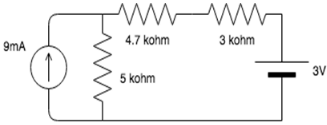Detailed Solution for Test: Source Transformations - Question 2

The 9mA source connected in parallel to the 5 kohm resistor can be converted to a 45V source in series with a 5 kohm resistor. Applying mesh analysis, we get:
I=(45-3)/(5+4.7+3)= 3.3mA.

Test: Source Transformations - Question 3

### Find the value of voltage once source transformation is applied to the circuit.​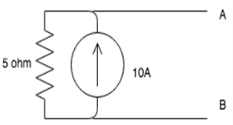Detailed Solution for Test: Source Transformations - Question 3

Using ohm’s law, we can use the relation: V=IR.
Thus V=10*5= 50V.

Test: Source Transformations - Question 4

Once the circuit is transformed to a voltage source where will the resistance be connected?​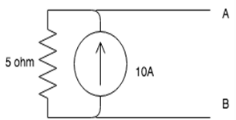Detailed Solution for Test: Source Transformations - Question 4

The resistance is connected in series with the voltage source because we are transforming a current source connected in parallel to a resistor to a voltage source connected in series with it.

Test: Source Transformations - Question 5

What will the value of the current be once source transformation is applied to the circuit?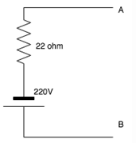Detailed Solution for Test: Source Transformations - Question 5

Using ohm’s law, we can use the relation: V=IR.
Thus I=V/R.
I=220/22=10A.

Test: Source Transformations - Question 6

Once the circuit is transformed to a current source where will the resistance be connected?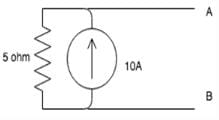Detailed Solution for Test: Source Transformations - Question 6

The resistance is connected in series with the voltage source because we are transforming a current source connected in parallel to a resistor to a voltage source connected in series with it.

Test: Source Transformations - Question 7

A current source connected in parallel with a resistor can be converted to a?

Detailed Solution for Test: Source Transformations - Question 7

A current source connected in parallel can be converted to a voltage source connected in series using the relation obtained from ohm’s law, that is V=IR. This equation shows that a current source connected in parallel has the same impact as a voltage source connected in series.

Test: Source Transformations - Question 8

A source transformation is_________

Detailed Solution for Test: Source Transformations - Question 8

A source transformation is bilateral because a voltage source can be converted to a current source and vice-versa.

Test: Source Transformations - Question 9

In source transformation________

Detailed Solution for Test: Source Transformations - Question 9

In source transformation, the value of the voltage and current sources change when changed from voltage to current source and current to voltage source but the value of the resistances remains the same.

Test: Source Transformations - Question 10

If there are 3 10V sources connected in parallel then on source transformation__________

Detailed Solution for Test: Source Transformations - Question 10

When voltages are connected in parallel, the effect of only one source is considered because the effect of the voltage remains the same when connected in parallel.

## Basic Electrical Technology

58 docs|59 tests
 Use Code STAYHOME200 and get INR 200 additional OFF Use Coupon Code
Information about Test: Source Transformations Page
In this test you can find the Exam questions for Test: Source Transformations solved & explained in the simplest way possible. Besides giving Questions and answers for Test: Source Transformations, EduRev gives you an ample number of Online tests for practice

58 docs|59 tests